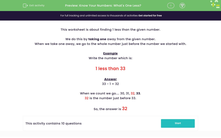# Know Your Numbers: What's One Less?

In this worksheet, students identify one number less than the given number (from a range between 0 and 100).Key stage:  KS 1

Curriculum topic:   Number: Number and Place Value

Curriculum subtopic:   Identify One More/One Less

Difficulty level:#### Worksheet Overview

This worksheet is about finding 1 less than the given number.

We do this by taking one away from the given number.

When we take one away, we go to the whole number just before the number we started with.

Example

Write the number which is:

1 less than 33

33 - 1 = 32

When we count we go.... 30, 31, 32, 33.

32 is the number just before 33.